# Pythagorean Triples

Pythagorean Triples
Go back to  'Geometry'

In general, historians believe that the first mathematician was Pythagoras of Samos (born circa 569 BC in Samos, Ionia, and died circa475 BC). He is an extremely important figure in mathematical progress, but his mathematical accomplishments are very little known.

In this mini-lesson, we will explore all about Pythagorean triples. We will understand what they mean, what are its general formulas, how to calculate it and discover interesting facts around them.

Look at the calculator below, and see how positive Integers 3, 4, and 5 satisfy the equation as Pythagorean triples. We will learn more about it in the coming sections.

You can check out the interactive examples to know more about the lesson and try your hand at solving a few interesting practice questions at the end of the page.

## Lesson Plan

 1 What Are Pythagorean Triples? 2 Important Notes on Pythagorean triples 3 Challenging Questions on Pythagorean triples 4 Solved Examples on Pythagorean triples 5 Interactive Questions on Pythagorean triples 6 Frequently Asked Questions (FAQs)

## What Are Pythagorean Triples?

Pythagorean triples are often defined as a group of three positive whole numbers that completely satisfy the Pythagorean theorem.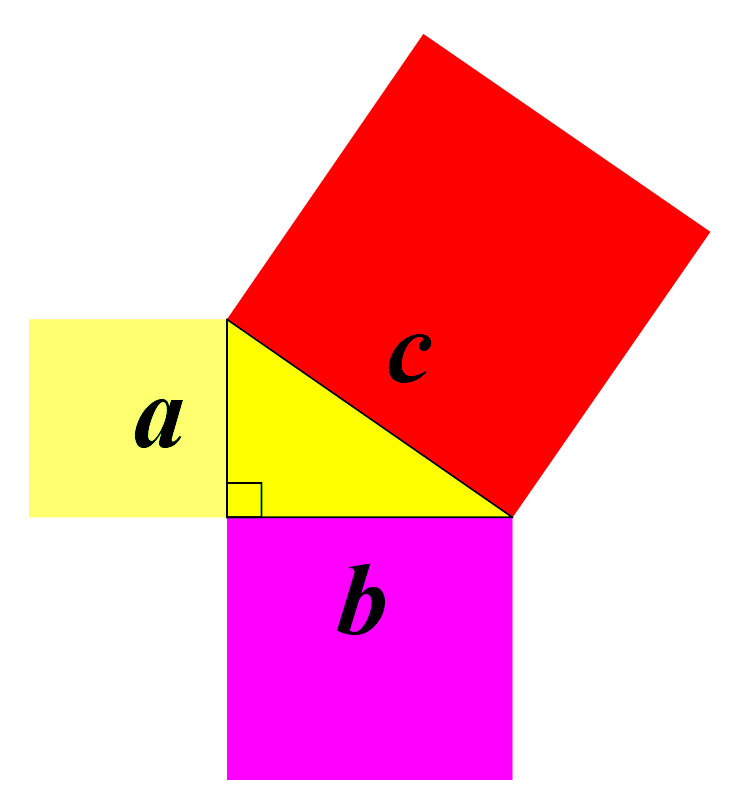$a^2 + b^2 = c^2$

Where a, b, and c are the Positive Integers that satisfy this equation as Pythagorean triples.

Look at the simulation below How Positive Integers  3, 4, and 5 satisfy the equation as Pythagorean triples.

## What Is the General Formula of Pythagorean Triples?

The general formula for Pythagorean triplet can be shown as,

 $a^2 + b^2 = c^2$
• Where 'c' is the "hypotenuse" on the longest side of the triangle.

• The other two sides are a and b

The most popular example of Pythagorean triples is 3, 4, and 5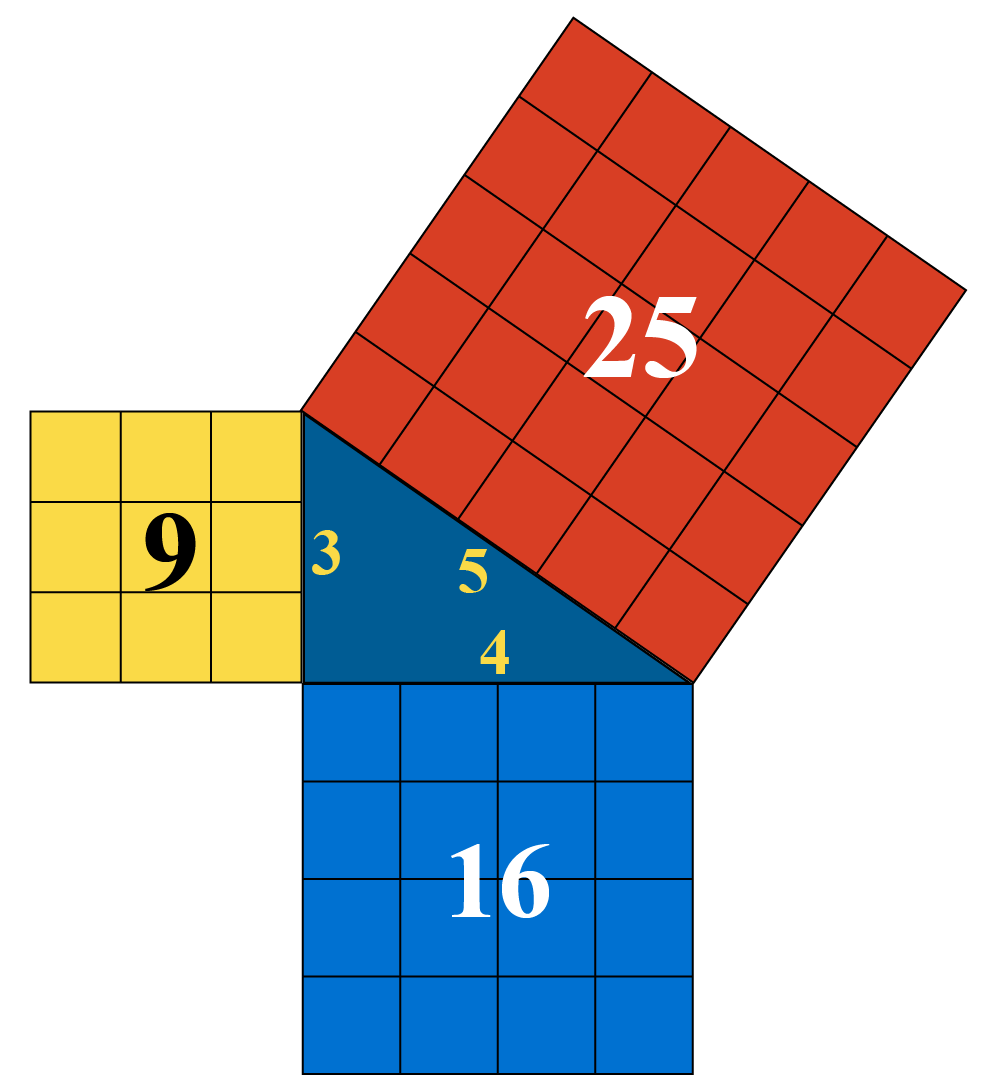The two more examples are: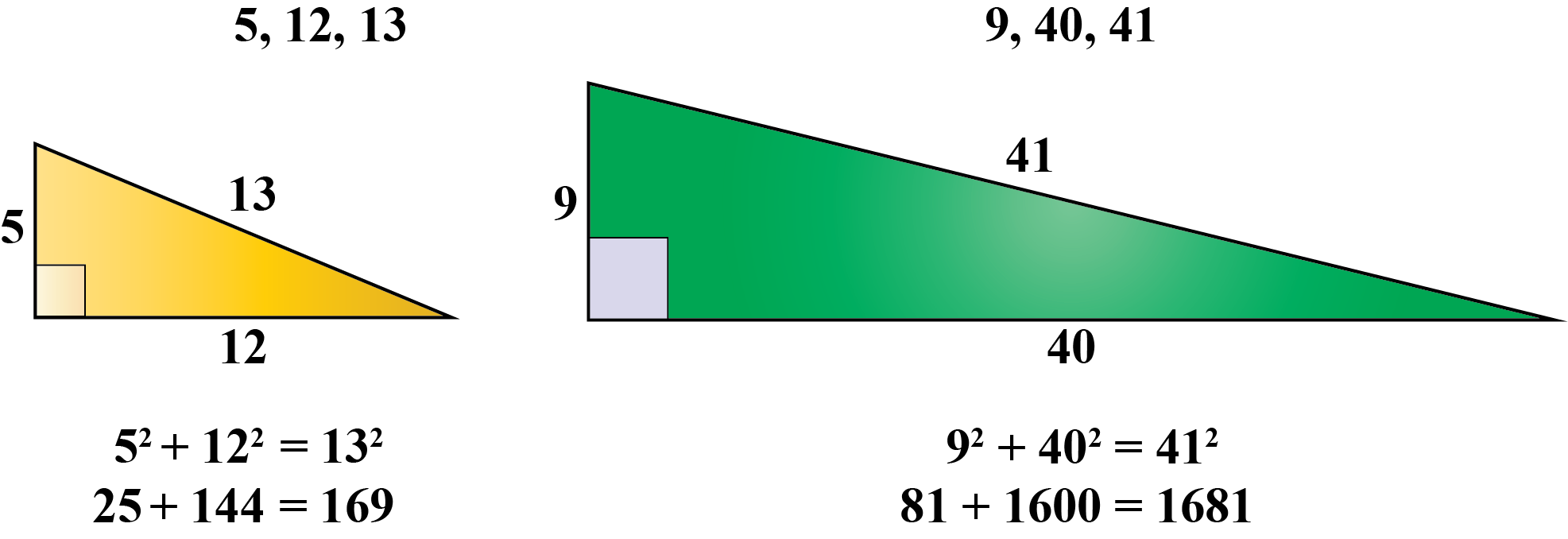The set of the Pythagorean triples is endless.

The first Pythagorean triple is (3, 4, and 5).

Let n be any integer than 1, then 3n, 4n, and 5n are also set of the Pythagorean triple.

This is true because  $${\left( {{\rm{3n}}} \right)^{\rm{2}}} + {\left( {{\rm{4n}}} \right)^{\rm{2}}} = {\left( {{\rm{5n}}} \right)^{\rm{2}}}$$

Examples:

n (3n, 4n, 5n)
2 (6, 8, 10)
3 (9, 12, 15)
4 (12, 16, 20)

Look at the simulation below for the Pythagorean triple generator.

It shows the Pythagorean triples of the multiples of (3n, 4n 5n).

Just drag the slider and obtain the Pythagorean triples list of the multiples of (3n, 4n, and 5n).

## What Are the Different Categories of Pythagorean Triplets?

There are two types of Pythagorean triples:

•    Primitive Pythagorean triples
•    Non-primitive Pythagorean triples

### Primitive Pythagorean triplets

A Primitive Pythagorean triple is a type of pythagorean triple which is a reduced set of the positive values of a, b, and c with a common factor other than 1. And it also satisfies the Pythagorean theorem.

$a^2 + b^2 = c^2$

Example 1

$\begin{array}{l} {\rm{(3, 4, 5) \text{ Its greatest common factor} = 1}} \\[0.2cm] {\rm{a^2 + b^2 = c^2}} \\[0.2cm] {\rm{3^2 + 4^2 = 5^2}} \\[0.2cm] {\rm{9 + 16 = 25}} \\[0.2cm] {\rm{25 = 25}} \\ \end{array}$

Example 2

$\begin{array}{l} {\rm{(5, 12, 13) \text{ Its greatest common factor} = 1}} \\[0.2cm] {\rm{a^2 + b^2 = c^2}} \\[0.2cm] {\rm{5^2 + 12^2 = 13^2}} \\[0.2cm] {\rm{25 + 144 = 169}} \\[0.2cm] {\rm{169 = 169}} \\ \end{array}$

### Non-Primitive Pythagorean triplets

A non-primitive triple Pythagorean, also known as the imperative triple Pythagorean, is a sequence of positive a, b, and c values with a general factor exceeding 1.

In other words, the three sets of positive values in a non-primitive Pythagorean triple are all even numbers.

Examples of non-primitive Pythagorean triples include: (6, 8, 10), (32, 60, 68), etc.

Example

(6, 8, 10)
$\begin{array}{l} {\rm{\text{Greatest common factor of 6, 8, and 10 } = 2}} \\[0.2cm] {\rm{a^2 + b^2 = c^2}} \\[0.2cm] {\rm{6^2 + 8^2 = 10^2}} \\[0.2cm] {\rm{36 + 64 = 100}} \\[0.2cm] {\rm{100 = 100}} \\ \end{array}$Important Notes
• Any three numbers which satisfy the formula of $$a^2 + b^2 = c^2$$ are known as Pythagorean triples.
• If any number of a Pythagorean triple is given, then the other two numbers can be generated by using, $$a = m^2 - n^2$$, b = 2mn & $$c = m^2 + n^2$$.
• Pythagorean triples can not be in decimals.

## Solved Examples

 Example 1

John's mother asked him to place the ladder at such a distance from the wall, such that the 13 feet tall ladder's head falls exactly on the top of the 12 feet wall.

Can you help John to find the distance of the ladder from the wall?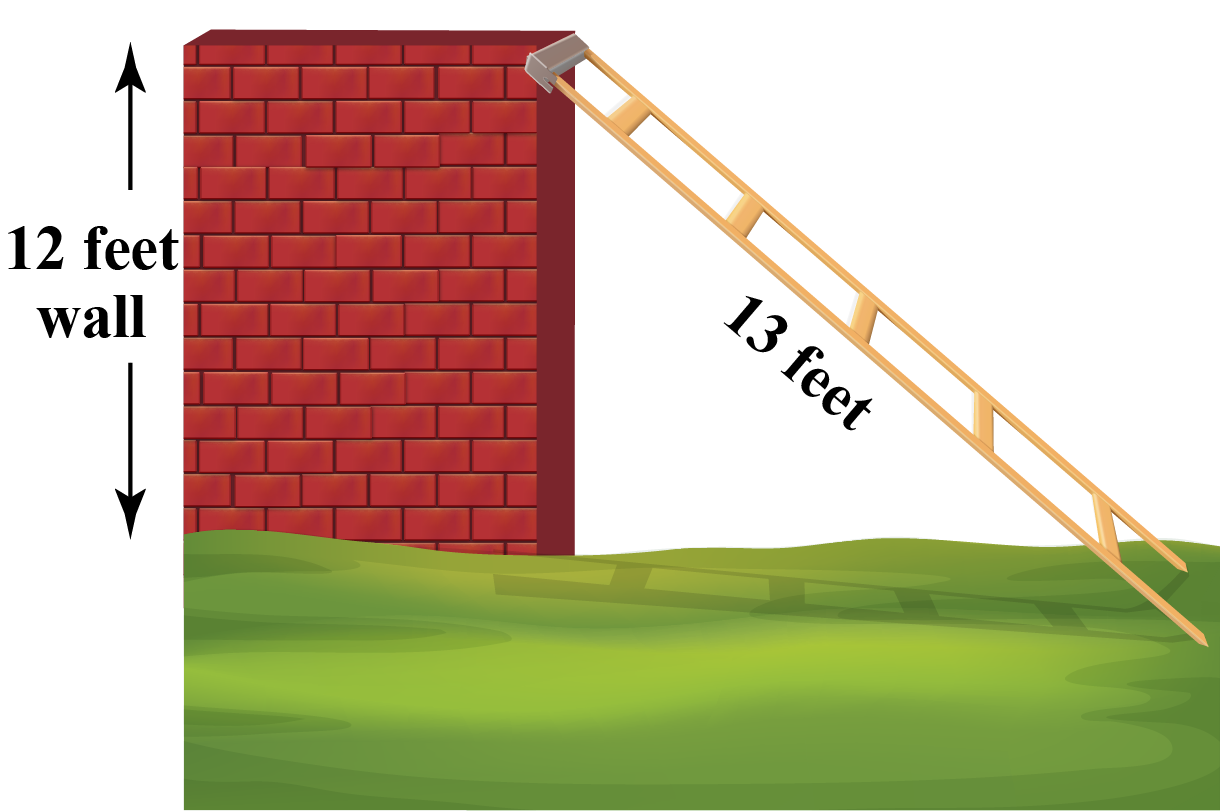Solution

Let the required distance be '$$x$$' feet.

Here, the ladder, the wall, and the ground form a right-angled triangle. The ladder is the hypotenuse of the triangle.

According to the Pythagorean theorem,

\begin{align} x^2 + 12^2 &= 13^2 \\[0.2cm] x^2 &= 13^2 - 12^2 \\[0.2cm] x^2 &= (13 - 12)(13 + 12) \\[0.2cm] x^2 &= (1)(25) \\[0.2cm] x &= 5 \\ \end{align}

 $\therefore \text{ The distance is 5 feet }$
 Example 2

Joey tried a new route to reach his school today. He walked 6 blocks to the north, and then 8 blocks to the west.

Can you find how far is his school from his home?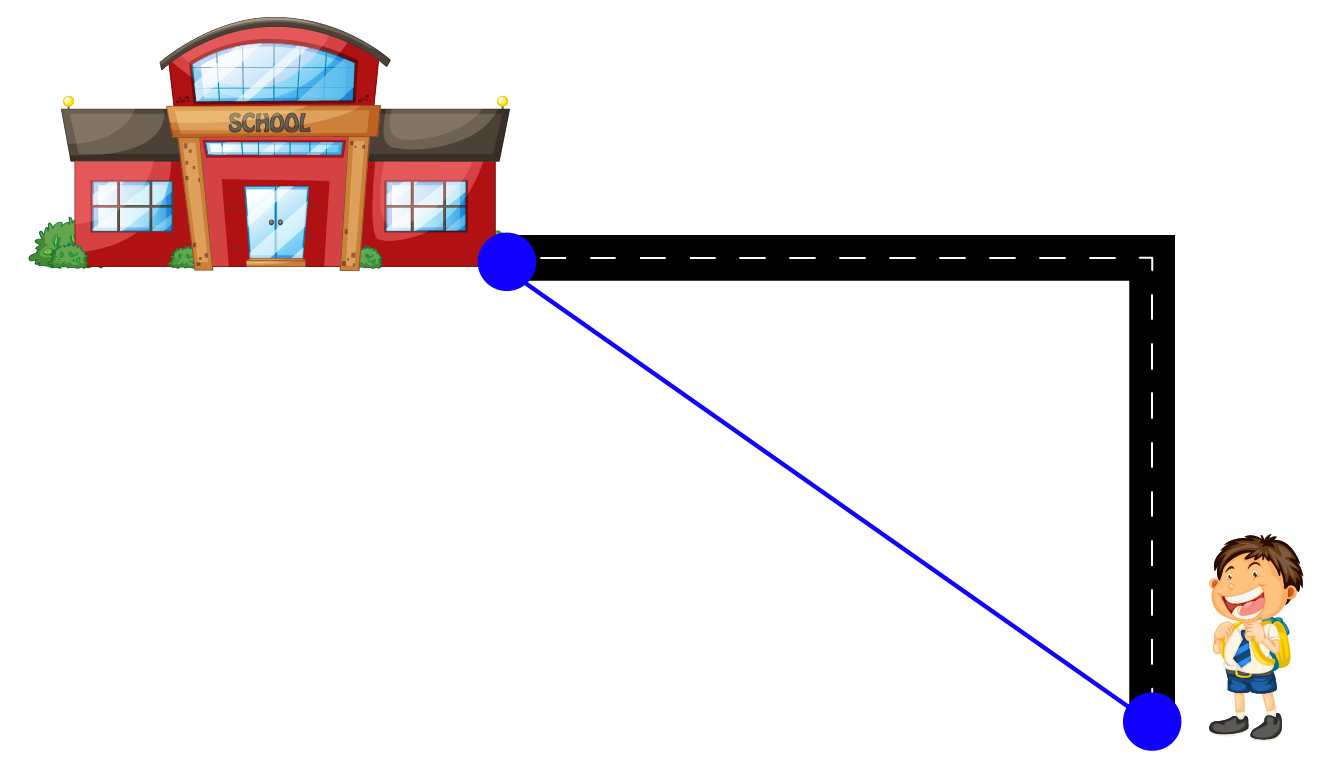Solution

The distance from the school to home is the length of the hypotenuse.

Let c be the missing distance from school to home and a=6, b=8

\begin{align} c^2&= a^2 + b^2 \\[0.2cm] c^2 &= 6^2 + 8^2 \\[0.2cm] c^2 &= 36 + 64 \\[0.2cm] c^2 &= 100 \\[0.2cm] c &= 10 \\[0.2cm] \end{align}

 $$\therefore$$The distance from school to home is 10 blocks.
 Example 3

Susan bought a new carpet, whose length is 7 feet, and the diagonal is $$\sqrt {74}$$ feet. Can you help her figure out the width of that carpet?

Solution

Let the width of the carpet = '$$x$$' feet.

\begin{align} (Diagonal)^2 &= (Length)^2 + (Width)^2 \\[0.2cm] (\sqrt {74} )^2 &= (7)^2 + x^2 \\[0.2cm] 74 &= 49 + x^2 \\[0.2cm] 74 - 49 &= x^2 \\[0.2cm] 25 &= x^2 \\[0.2cm] x &= 5 \\ \end{align}

 $\therefore \text{ The width of the carpet is 5 feet }$
 Example 4

Jim and Jill start cycling from the same destination together. They both reach different locations, which are $$17\sqrt 2$$ miles apart from each other. Can you find how much distance did each travel?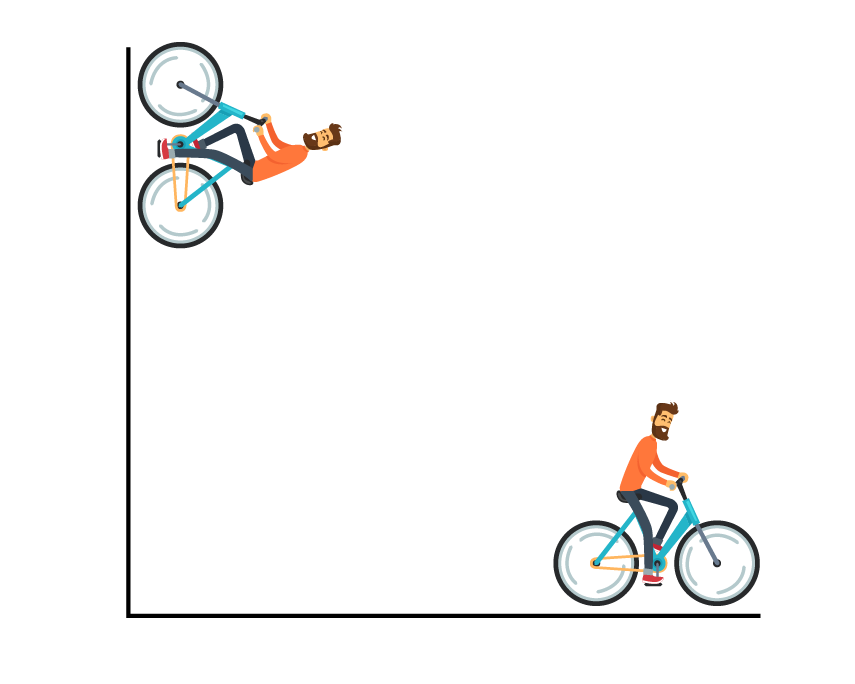Solution

\begin{align} x^2 + x^2 &= (17\sqrt 2 )^2 \\[0.2cm] 2x^2 &= 289\times 2 \\[0.2cm] x &= 17 \\ \end{align}

 $$\therefore$$The distance is 17 miles
 Example 5

The height of the building is 34 feet and 29 feet respectively. Can you find the difference in the heights of their tops if the distance AC is known to be 12 feet?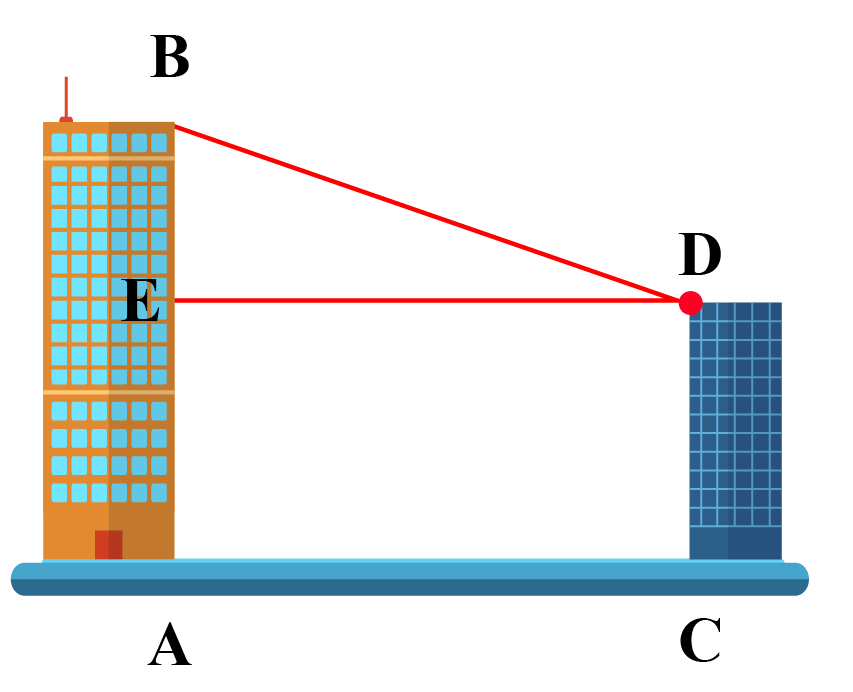Solution

Draw $${\rm{DE}} \bot {\rm{AB}}$$

Then AE = AB - EB, but EB = BC

Therfore, AE = 34 - 29 = 5 feet

Now, AED is a right-angled triangle and right-angled at E.

Therefore,

\begin{align} AD^2 &= AE^2 + ED^2 \\[0.2cm] AD^2 &= 5^2 + 12^2 \\[0.2cm] AD^2 &= 25 + 144 \\[0.2cm] AD^2 &= 169 \\[0.2cm] AD &= 13 \\ \end{align}

 $$\therefore$$ The distance between their tops is 13 feet.Challenging Questions
• A cube has a side length of 10 inches. What is the length of the diagonal through its center?

## Interactive Questions

Here are a few activities for you to practice.

## Let's Summarize

The mini-lesson targeted the fascinating concept of Pythagorean triples. The math journey around Pythagorean triples started with what a student already knew about Pythagoras theorem and went on to creatively crafting a fresh concept in the young minds. Done in a way that not only it is relatable and easy to grasp, but also will stay with them forever.

At Cuemath, our team of math experts is dedicated to making learning fun for our favorite readers, the students!

Through an interactive and engaging learning-teaching-learning approach, the teachers explore all angles of a topic.

Be it worksheets, online classes, doubt sessions, or any other form of relation, it’s the logical thinking and smart learning approach that we, at Cuemath, believe in.

## 1. What is the Pythagorean triples of 6?

The Pythagorean triples for 6 are (6, 8, 10).

## 2. Can Pythagorean triples have Decimals?

Pythagorean triples are the positive integers that fit the formula for the Pythagorean theorem. These are natural numbers that thus, can't be decimal.

## 3. What are the 5 most common Pythagorean triples?

The 5 most common Pythagorean triples are (3, 4, 5), (5, 12, 13), (6, 8, 10), (9, 12, 15), and (15, 20, 25)

Geometry
Pythagorean Triples
Finding the 3 sides of a right triangle

More Important Topics
Numbers
Algebra
Geometry
Measurement
Money
Data
Trigonometry
Calculus